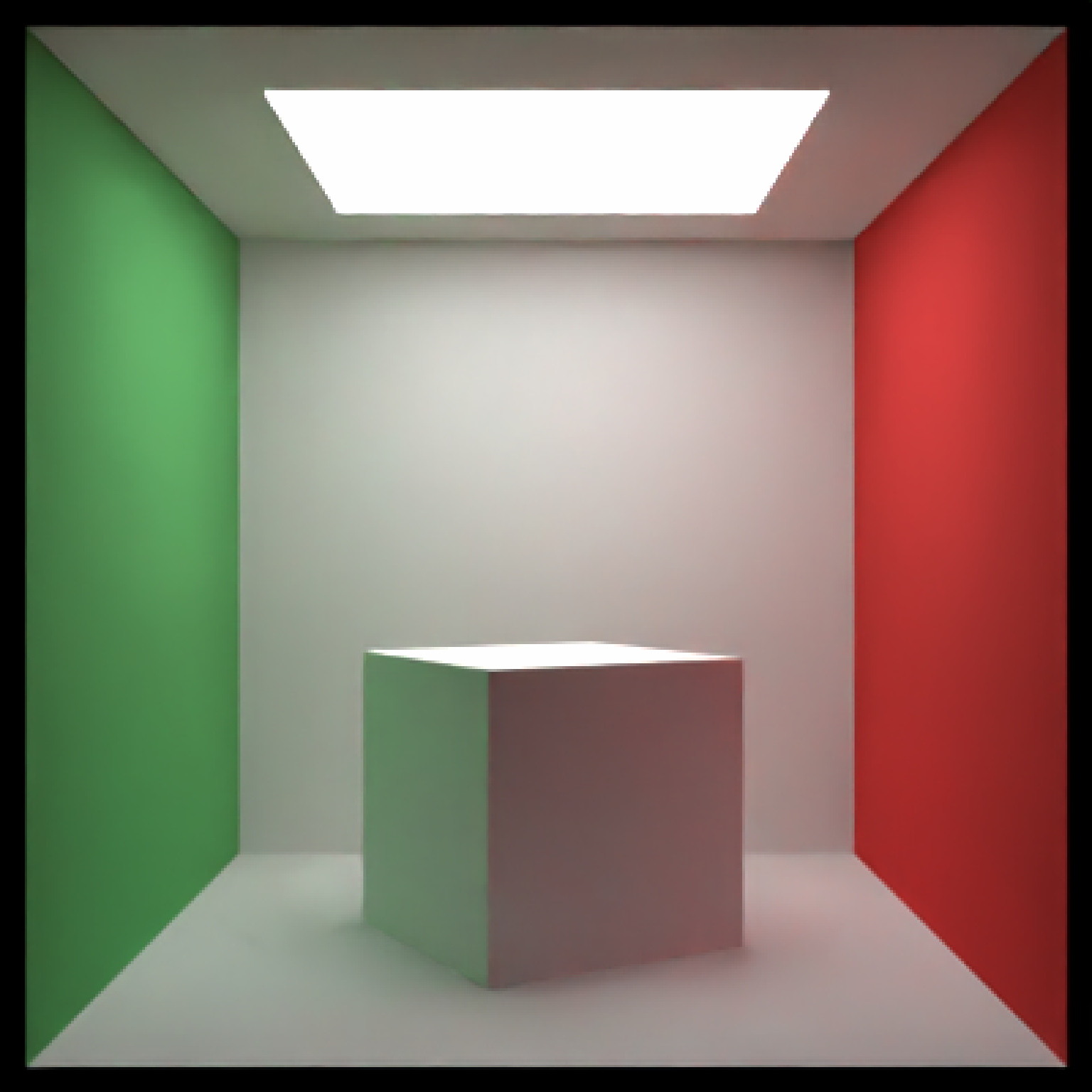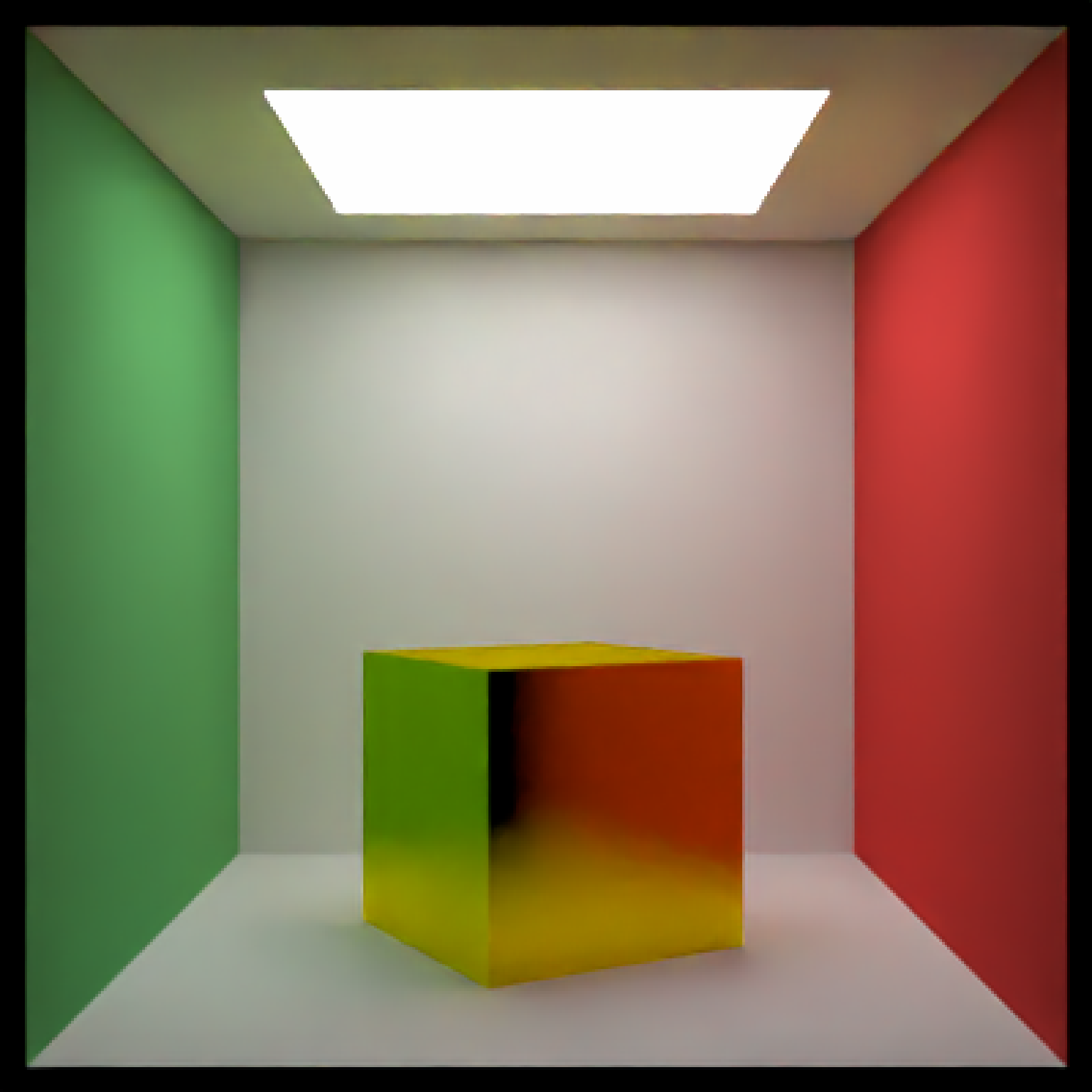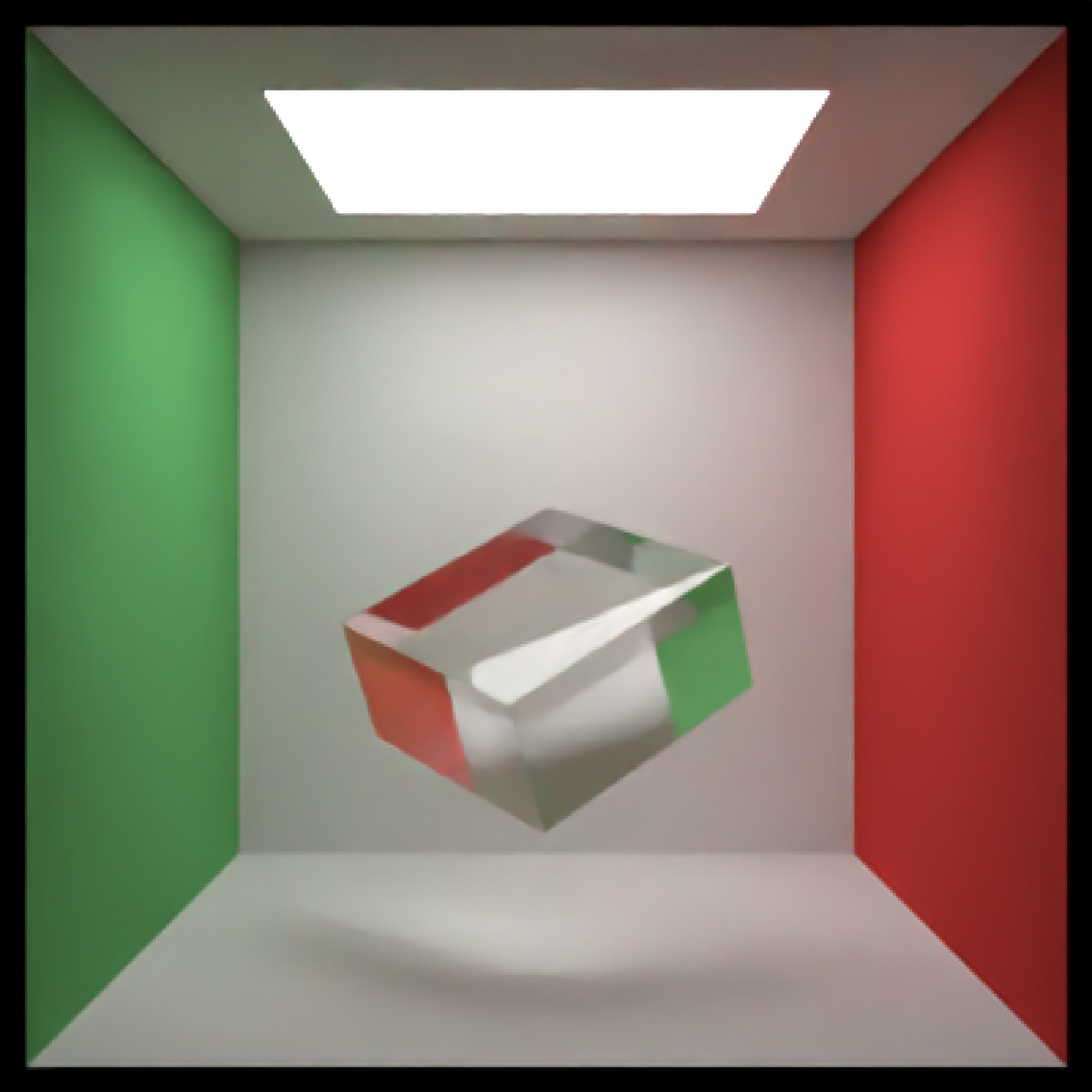Cube Object

cube(
x = 0,
y = 0,
z = 0,
width = 1,
xwidth = 1,
ywidth = 1,
zwidth = 1,
material = diffuse(),
angle = c(0, 0, 0),
order_rotation = c(1, 2, 3),
flipped = FALSE,
scale = c(1, 1, 1)
)

## Arguments

x

Default 0. x-coordinate of the center of the cube

y

Default 0. y-coordinate of the center of the cube

z

Default 0. z-coordinate of the center of the cube

width

Default 1. Cube width.

xwidth

Default 1. x-width of the cube. Overrides width argument for x-axis.

ywidth

Default 1. y-width of the cube. Overrides width argument for y-axis.

zwidth

Default 1. z-width of the cube. Overrides width argument for z-axis.

material

Default diffuse.The material, called from one of the material functions diffuse, metal, or dielectric.

angle

Default c(0, 0, 0). Angle of rotation around the x, y, and z axes, applied in the order specified in order_rotation.

order_rotation

Default c(1, 2, 3). The order to apply the rotations, referring to "x", "y", and "z".

flipped

Default FALSE. Whether to flip the normals.

scale

Default c(1, 1, 1). Scale transformation in the x, y, and z directions. If this is a single value, number, the object will be scaled uniformly. Note: emissive objects may not currently function correctly when scaled.

## Value

Single row of a tibble describing the cube in the scene.

## Examples

#Generate a cube in the cornell box.
if(run_documentation()) {
generate_cornell() %>%
add_object(cube(x = 555/2, y = 100, z = 555/2,
xwidth = 200, ywidth = 200, zwidth = 200, angle = c(0, 30, 0))) %>%
render_scene(lookfrom = c(278, 278, -800) ,lookat = c(278, 278, 0), fov = 40,
ambient_light = FALSE, samples = 128, parallel = TRUE, clamp_value = 5)
}#Generate a gold cube in the cornell box
if(run_documentation()) {
generate_cornell() %>%
add_object(cube(x = 555/2, y = 100, z = 555/2,
xwidth = 200, ywidth = 200, zwidth = 200, angle = c(0, 30, 0),
material = metal(color = "gold", fuzz = 0.2))) %>%
render_scene(lookfrom = c(278, 278, -800) ,lookat = c(278, 278, 0), fov = 40,
ambient_light = FALSE, samples = 128, parallel = TRUE, clamp_value = 5)
}#Generate a rotated dielectric box in the cornell box
if(run_documentation()) {
generate_cornell() %>%
add_object(cube(x = 555/2, y = 200, z = 555/2,
xwidth = 200, ywidth = 100, zwidth = 200, angle = c(-30, 30, -30),
material = dielectric())) %>%
render_scene(lookfrom = c(278, 278, -800) ,lookat = c(278, 278, 0), fov = 40,
ambient_light = FALSE, samples = 128, parallel = TRUE, clamp_value = 5)
}